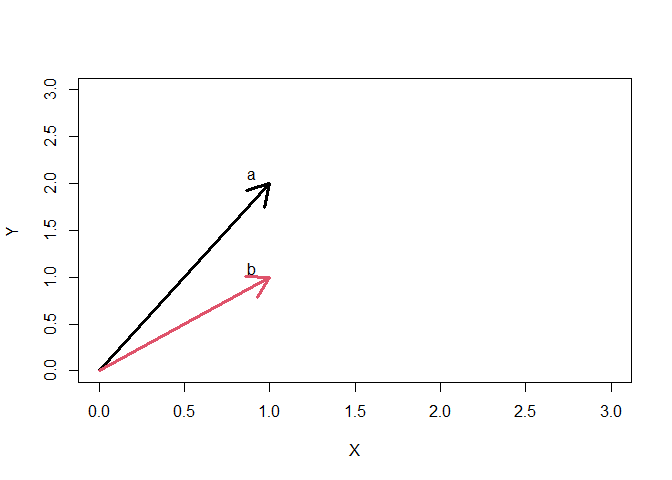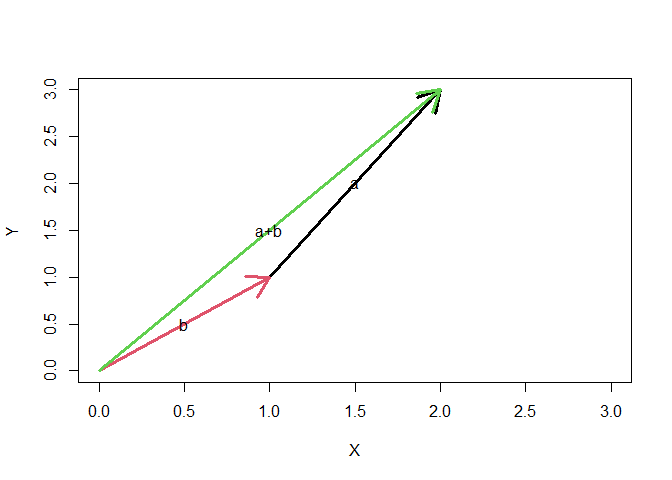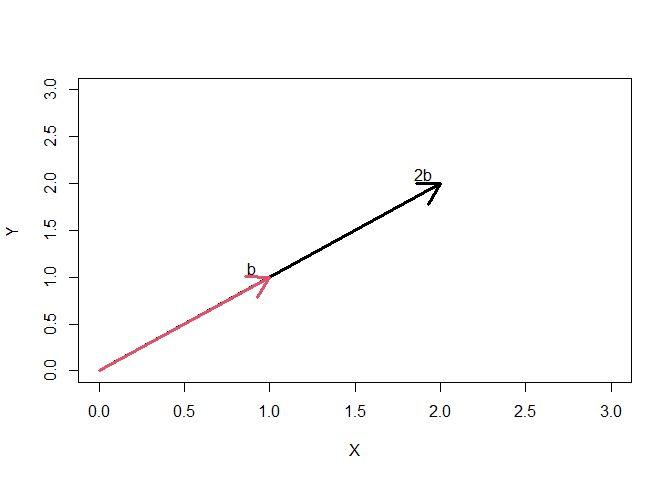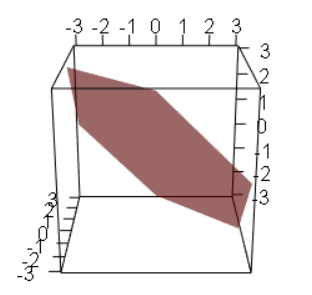# Vectors (R)

## Basics of vectors¶

Consider two vectors a and b

a <- c(1,2)
b <- c(1,1)


The two vectors can be visualised in a 2D coordinate system as follows:We can perform two types of linear operations on these vectors:
2. Multiplication of the vector with a scalar

If we add a and b together, the sum would be a vector whose members are the sum of the corresponding members from a and b.

a+b

##  2 3Vector multiplication: If we multiply b by 2, we will get a vector with each of its members multiplied by 2.

2*b

##  2 2## Subspaces and span¶

As we can perform only two kinds of linear operations on the vectors, any linear combinations will be of the form:
$$S= \alpha a + \beta b$$ Where $\alpha$ and $\beta$ are real numbers and $a$ and $b$ are vectors.

What about all possible linear combinations of $a$ and $b$?The linear combination of the vector a and b form the entire 2D plane.

Vector space is the space in which the vector can exist. A 2D vector, like a or b, will have vector space of $R^2$ and a 3D vector like d=[1,2,3] is in the vector space $R^3$.
Span: The set of all possible linear combinations of vectors is called the span of those set of vectors.

For the above two vectors a = [1,2] and b=[1,1], the entire 2d space is the span, as we can get every vector in the 2d space as a linear combination of the two vectors.

To explain the difference between vector space and vector span, consider the two vectors d=[1,2,3] and e=[1,1,1] As the two vectors are in 3 dimensions, they have a vector space of 3 or $R^3$.
Adding d+e I get another vector in 3d.

d <- c(1,2,3)
e <- c(1,1,1)
d+e

##  2 3 4


But what are all the linear combinations for d and e?This forms a 2d plane which goes thru the origin. Therefore these vectors span a plane (in $R^2$) although their vector space is ($R^3$).The maximum span that any set of vectors can have is equal to their vector space.

## Linear Independence¶

Linear independence is when one vector has no relationship with another. In the first example with a=[1,2] and b=[1,1], any vectors in the 2d space can be written as a linear combination of a and b. In the second example with d=[1,2,3] and e=[1,1,1], any vector on the plane can be written as a linear combination of d and e. A vector which is not in the plane, like f = [2,3,3] is linearly independent of d and e, as no $\alpha$ and $\beta$ satisfy $f=\alpha d+\beta e$.

In a vector space of n dimensions (vector space is n), there can be at max n vectors which are linearly independent.

## Bases, norms and inner products¶

A basis for $R^n$ space is any linearly independent set of vectors S such that span(S) = n.
From the above examples, a and b are in the vector space $R^2$ and also have their span as $R^2$. Therefore they form a basis for $R^2$.
Similarly, the three independent vectors d, e and f are in the vector space $R^3$ and form a basis for $R^3$.

The standard basis for $R^2$ is [1.0] and [0,1].

The norm of the vector is the length of the vector.

$l_2 \,norm(\bar{v}) = \sqrt{x_1^2 + y_1^2+..}$
norm(a,  type="2")

##  2.236068

norm(d,  type="2")

##  3.741657


The dot product (or inner product) takes two vectors as an input and returns a number as an output. It is defined as $\bar{x}.\bar{y} = \sum{x_i\times y_i}$. It represents the length of the shadow of one vector on the other.

library(geometry)
dot(a,b)

##  3


In the next post in this series, I will talk about matrices.